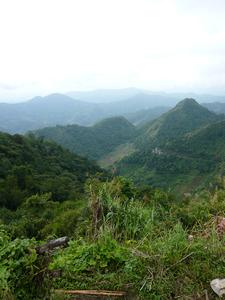Related Articles

# Calculate the area of an image using Matplotlib

• Last Updated : 31 Jul, 2021

Let us see how to calculate the area of an image in Python using Matplotlib.

Algorithm:

1. Import the matplotlib.pyplot module.
2. Import an image using the imread() method.
3. Use the shape attribute of the image to get the height and width of the image. It fetches the number of channels in the image.
4. Calculate the area as, area = height * width.
5. Display the area.

Example 1: Consider the following image :“GFG.jpg”

## Python3

 `# import necessary library` `import` `matplotlib.pyplot as plt`   `# read an image` `img ``=` `plt.imread(``"GFG.jpg"``)`   `# fetch the height and width` `height, width, _ ``=` `img.shape`   `# area is calculated as “height x width”` `area ``=` `height ``*` `width`   `# display the area` `print``(``"Area of the image is : "``, area)`

Output :

`Area of the image is : 50244`

Example 2: Consider the following image :“image.jpg”

## Python3

 `# import necessary library` `import` `matplotlib.pyplot as plt`   `# read an image` `img ``=` `plt.imread(``"image.jpg"``)`   `# fetch the height and width` `height, width, _ ``=` `img.shape`   `# area is calculated as “height x width”` `area ``=` `height ``*` `width`   `# display the area` `print``(``"Area of the image is : "``, area)`

Output :

`Area of the image is : 213200`

Attention geek! Strengthen your foundations with the Python Programming Foundation Course and learn the basics.

To begin with, your interview preparations Enhance your Data Structures concepts with the Python DS Course. And to begin with your Machine Learning Journey, join the Machine Learning – Basic Level Course

My Personal Notes arrow_drop_up
Recommended Articles
Page :